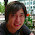## Monday, February 4, 2013

### Conditional Random Fields: I finally got it!

As a combination of trying again and again and the classes I take I was able to wrap my head around why CRFs are great and how they work. At first I was a bit sceptic since I love my HMMs but maybe in the future I give it a try. I think the main reason I got what these models do is that there is a very deep discussion on Markov Random Fields in our Probabilistic Graphical Models lecture (by Jim Rehg) CS 8803 at Georgia Tech. Since I will go a bit deeper in the model itself in a second here is the important bit. The first difference is that Random Fields are a distribution over potentials which are just any positive function defined over a clique of variables in the model. The joint probability is then defined as a scaled multiplication of these potentials. In Hidden Markov Models we have a graph structure over probability distributions instead. The difference of a Conditional Random Field to a Markov Random Field is that it models the conditional probability instead of the joint probability. The only difference however seems to be the definition of the scaler.

Now to the details. In contrast to a Bayes Network or a Hidden Markov Model a Random field is a undirected model. So instead of talking about a parent child relationship we talk about interaction between potentials. A potential is a function defined over variables of a clique. Just as a reminder,
in Computer Science a clique is a fully connected subgraph. For example if we were to label each frame in a sequence we could use the model below. The Y are the labels over time and X is the whole time series.

The potential function is defined as a three- clique over the last label, the current label and the whole time series.

While the transition probability (label sequence) is the same as in Hidden Markov Models as it models a
first order Markov Chain, the observations are fully accessible. In a Hidden Markov Model the observations have to be independent by definition. So now can define more powerful observations by designing potential functions that take previous values into account. Furthermore, we can use the labels in order to behave differently from label to label. The joint probability is then defined over these potentials. However for labeling the sequence we are not interested in the joint probability but in the conditional as P(Y | X) instead of P(Y, X). The conditional probability is then defined as:

And this is a Conditional Random Field. In my opinion and from what I read, this model is more powerful then Hidden Markov Models but has some drawbacks. The first one is that in order to train it, we need fully labeled data. While in a Hidden Markov Model, we can automatically distribute the data over the states by inferring the label sequence, this is not possible here. The reason is that instead of a Expectation Maximisation training, most people seem to use a Gradient Based optimization on the log-likelihood. Acquiring labels on that level might not be possible in all cases. The other thing I read is that these models need a lot more training data for training and the training is much slower. In the end that means use cases where only a few training instances can be collected this model won't be able to converge.

1.Thank you Daniel Kohlsdorf , I was facing the same problem before reading this... thanks again

2.Cool :) thanks! You might find interesting the free framework of https://recast.ai (a sweet AI collaborative platform which allows developers to build bots which understand natural languages (NLP) in minutes! check it out ;) thanks again for this super clear post!

3.The observations don't have to independent in the HMM, if they are not then it just becomes auto-regressive HMM (see page 626 of Murphy).

4.Well yes there are hmms where the observations are not independent. Thanks for the pointer!
However, in the classic case they are, which I talk about here for simplicity.